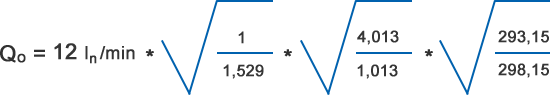Alue & kieli: Suomi / Suomi
Jälleenmyyjien kirjautuminen# Gas flow meter calculation formulas

Here you can find calculation formulas for gas flow conversions. Use the conversion formula shown below. Here’s also few examples to help you!

### Gas flow conversion formula### Actual flow rate

Q0 = Actual flow rate

ρ0 = Specific gravity of measured gas (Air = 1)

p0 = Actual ABSOLUTE pressure in metering tube

T0 = Actual ABSOLUTE temperature
(Kelvin) in metering tube

(0°C = 273,15°K)
(20° C = 293,15°K)

### Flowmeter design (calibration) conditions

ρ1 = Specific gravity of design gas (Air = 1)

p1 = ABSOLUTE design pressure
(calibration pressure)

T1 = ABSOLUTE design temperature
(Calibration temperature)

## Example 1

What is the maximum flow if flow meter EK-3NR is used for Carbon dioxide at 3 bar(g) / 25°C.

Ex-3NR scale is

• 1 – 12 ln/min Air at 0 bar(g)/ 20°C

Q1 = 12 ln/min
ρ1 = 1
p1 = (0 + 1,013) bar = 1,013 bar
T1 = 293,15° K

Q0 = ?
ρ0 = 1,529
p0 = (3+ 1,013) bar = 4,013 bar
T0 = 298,15° KActual flow at 12 ln/min reading
Q0 = 19,15 ln/min CO2 at 3 bar(g)/ 25°C

## Example 2

What is the actual flow if flow meter LH-JJ32 is used for Helium at 5 bar(g) / 10°C.

LH-JJ32 is calibrated for

• 30 ln/min Nitrogen at 3 bar(g)/ 20°C

Q1 = 30 ln/min
ρ1 = 0,9672
p1 = (3 + 1,013) bar = 4,013 bar
T1 = 293,15°K

Q0 = ?
ρ0 = 0,1380
p0 = (5+ 1,013) bar = 6,013 bar
T0= 283,15°KActual flow at 30 ln/min reading
Q0 = 98,9 ln/min He at 5 bar(g)/ 10°C

### Suomi Pääkonttori

Kytola Instruments Oy
PL 5
Olli Kytölän tie 1
40951 Muurame

Hidden
Hidden
Kenttä on validointitarkoituksiin ja tulee jättää koskemattomaksi.